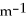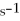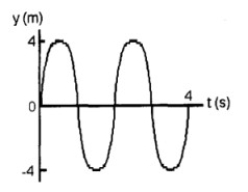# Physics Study Set 2

Physics & Astronomy

## Quiz 14 :Waves and SoundLooking for Introductory Physics Homework Help?

## Quiz 14 :Waves and Sound

Showing 1 - 20 of 104Give at least one example of each of the following: (a) longitudinal standing wave. (b) transverse standing wave.
Free
Essay

(a)sound wave resonating in an organ pipe
(b)vibrating string on a violinA uniform rope hangs from a ceiling. Without tugging on the rope, you give the bottom of the rope a wiggle and a wave travels upward along the rope. What can you say about the speed of the wave as the wave travels up the rope?
Free
Essay

The speed increases because the tension is increasing.Write an expression for a harmonic wave that has an amplitude of 18 cm, a wavelength of 2.0 m, and propagates to the left at 15 m/s.
Free
Essay

y(x,t)= (18 cm)cos[(3.1)x + (47)t] or
y(x,t)= (18 cm)cos[((2π/2.0))x + ((2π × 7.5))t]What is the audible range of sound frequencies?In western movies you often see a person putting his ear against the rails in the train tracks to find out if a train is approaching. Why would this give him advance knowledge of the approach of a train?
EssaySound waves in air are transverse waves.
True FalseA wave pulse on a string whose end is fixed is reflected without inversion.
True FalseBats use infrasound to navigate.
True FalseInfrasound carries over long distances better than ultrasound.
True FalseFIGURE 14-1-In Figure 14-1, the wavelength is
Multiple ChoiceFIGURE 14-1-In Figure 14-1, the frequency is
Multiple ChoiceA wave pulse traveling to the right along a thin cord reaches a discontinuity where the rope becomes thicker and heavier. What is the orientation of the reflected and transmitted pulses?
Multiple ChoiceTwo strings are made of the same material. String 1 has radius r1 while string 2 has radius r2 = r1/2. When stretched by the same tension, the respective speed of propagation of waves v1 and v2 satisfy the condition
Multiple ChoiceFour waves are described by the following expressions, where distances are measured in meters and times in seconds. I y = 0.12 cos(3x - 21t) II y = 0.15 sin(6x + 42t) III y = 0.13 cos(6x + 21t) IV y = -0.23 sin(3x - 42t) Which of these waves travel in the +x direction?
Multiple ChoiceFour waves are described by the following expressions, where distances are measured in meters and times in seconds. I y = 0.12 cos(3x - 21t) II y = 0.15 sin(6x + 42t) III y = 0.13 cos(6x + 21t) IV y = -0.23 sin(3x - 42t) Which of these waves have the same speed?
Multiple ChoiceFour waves are described by the following expressions, where distances are measured in meters and times in seconds. I y = 0.12 cos(3x - 21t) II y = 0.15 sin(6x + 42t) III y = 0.13 cos(6x + 21t) IV y = -0.23 sin(3x - 42t) Which of these waves have the same period?
Multiple ChoiceAs the temperature of the air increases, what happens to the velocity of sound? (Assume that all other factors remain constant.)
Multiple ChoiceThe pitch of a sound is determined by
Multiple Choice